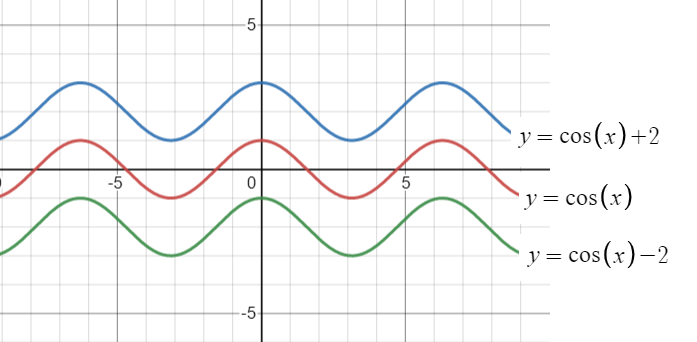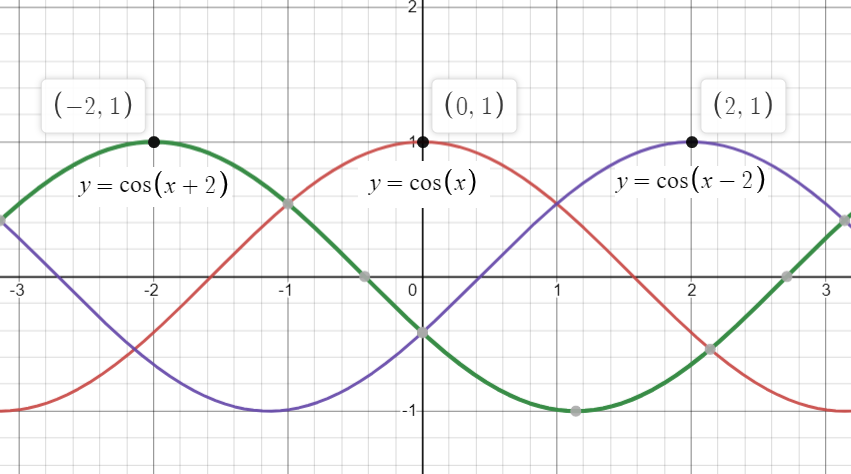# How to Translate a Function’s Graph

Two types of translation are possible for a function’s graph: shifting and scaling. You can also reflect a graph over the x-axis (or the y-axis); reflections are a special case of scaling, where x- or y-values are multiplied by a negative number.

## How to Translate a Function’s Graph with Shifts

Shifts, which change location but not shape, can be horizontal or vertical:

• Vertical translation: a shift up and down the y-axis. These shifts add or subtract a constant to every y-coordinate. For example, to move a graph up two units, add 2 to the function; to move the graph down, subtract 2.The graph of y = cos(x) (red) is shifted vertically by adding 2 (blue) or subtracting 2 (green).
• Horizontal translation: a shift left or right along the x-axis. These shifts add or subtract a constant to every x-coordinate. For example, to shift a graph left two units, add 2; to shift the graph right two units, subtract 2.The graph of y = cos(x) is shifted left or right by adding or subtracting 2 from the x-values.

## How to Translate a Function’s Graph with Scaling

Scaling alters the size and shape of a function’s graph by multiplying or dividing the function by a constant. This results in shrinks or stretches that can be horizontal or vertical:

• Vertical scale: Multiply or divide every y-coordinate by a constant.
• Horizontal scale: Multiply or divide every x-coordinate by a constant.

If the absolute value of the constant is less than 1, the graph will shrink. If the absolute value of the constant is greater than 1, the graph will stretch.The graph of y = cos(x) (red) is shrunk by multiplying by .3 (green) and stretched by multiplying by 2 (black).

## How to Translate a Function’s Graph with Reflections

Reflections are a special type of scaling where the graph is multiplied by a negative number. Multiplying the graph by -1 will reflect the graph without changing the shape, but you can multiply by another number to shrink or stretch the graph as well. For examples, see: Reflection Over The X-Axis.

## References

Graphs: Desmos.com.

CITE THIS AS:
Stephanie Glen. "How to Translate a Function’s Graph" From StatisticsHowTo.com: Elementary Statistics for the rest of us! https://www.statisticshowto.com/how-to-translate-a-functions-graph/